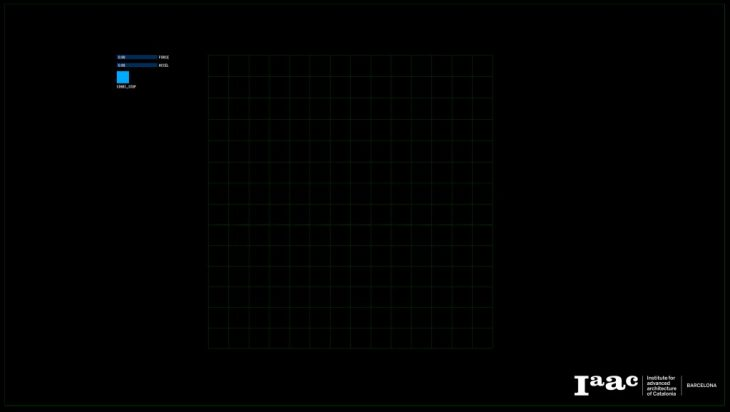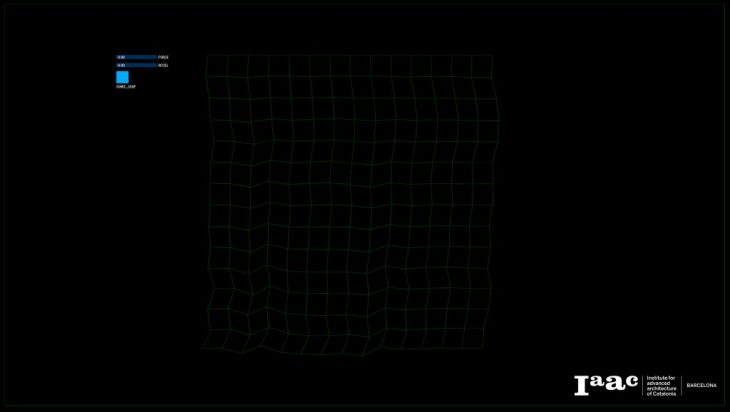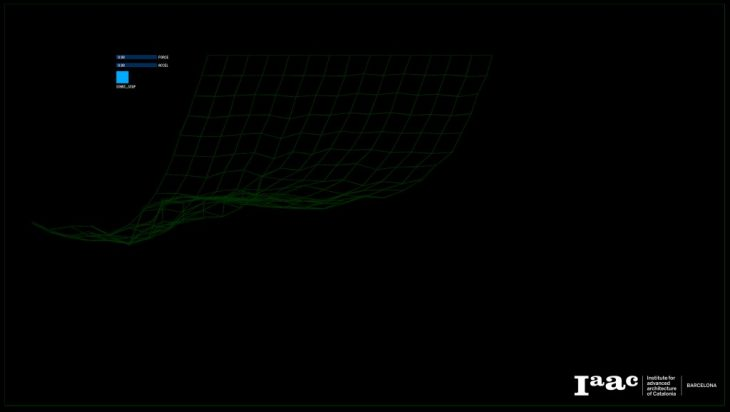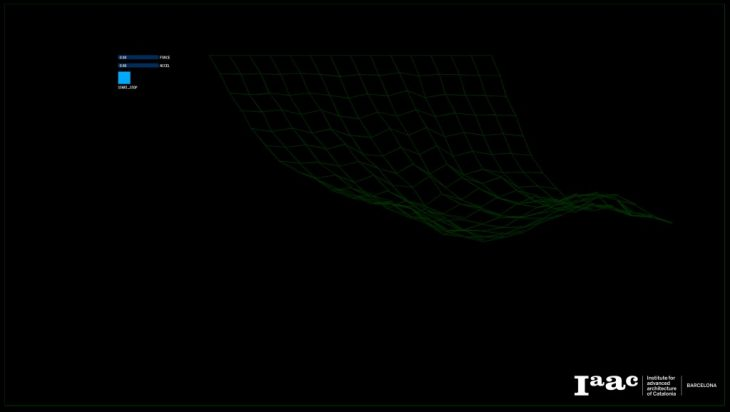Verlet integration is frequently used to calculate trajectories of particles in simulations and computer graphics. forces between points may be for example potentials constraining them to a specific distance. They may be considered as springs connecting the particles. Using springs of infinite stiffness, the model may then be solved with a Verlet algorithm.Verlet integration is useful because it directly relates the force to the position, rather than solving the problem via velocities. Problems, however, arise when multiple constraining forces act on each particle. One way to solve this is to loop through every point in a simulation so that at every point the constraint relaxation of the last is already used to speed up the spread of the information.                                                                     This algorithm is an attempt to contemplate between logic to physical calculations meanwhile preserving the canonical transformation of the system.Tutor: Angelos Chronis